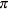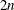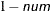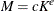The TIMESERIES Procedure

SPECTRA Statement

Subsections:
• SPECTRA statistics < / options>;

A SPECTRA statement can be used with the TIMESERIES procedure to specify which statistics appear in the OUTSPECTRA= data set. The SPECTRA statement options are used in performing a spectral analysis on the variables listed in the VAR statement. These options affect values that are produced in the PROC TIMESERIES statement’s OUTSPECTRA= data set, and in the periodogram and spectral density estimate. Only one SPECTRA statement is allowed.

The following univariate frequency domain statistics are available:

FREQ

frequency in radians from 0 toPERIOD

period or wavelength

COS

cosine transform

SIN

sine transform

P

periodogram

S

spectral density estimates

If none of the frequency domain statistics are specified, the default is as follows:

   spectra period p;


The following options can be specified in the SPECTRA statement following the slash (/):

C=coefficient

specifies the scale coefficient for the kernel function. See the section Kernel Option Details for more information.

E=exponent
EXP=exponent
EXPON=exponent

specifies the exponent for the kernel function. See the section Kernel Option Details for more information.

CENTER=NO | YES

specifies whether or not the series should be adjusted by its mean prior to performing the Fourier decomposition. This sets the first periodogram ordinate to 0 rather than totimes the squared mean. This option is commonly used when the periodograms are to be plotted to prevent a large first periodogram ordinate from distorting the scale of the plot. ADJUSTMEAN=YES specifies that the series be transformed by subtracting its mean. ADJUSTMEAN=NO specifies that no adjustment of the series be performed. The default is ADJUSTMEAN=NO.

ALPHA=num

specifies the width of a window that is drawn around the spectral density estimate in a spectral density versus frequency plot. Based on approximations proposed by Brockwell and Davis (1991), periodogram ordinates fall within this window with a confidence level of. The valuemust be between 0 and 1; the default is 0.5.

DOMAIN=domain

specifies how the smoothing function is interpreted. You can specify the following domain values:

FREQUENCY

smooths the periodogram ordinates.

TIME

applies the kernel as a filter to the time series autocovariance function.

By default DOMAIN=FREQUENCY, and smoothing is applied in the same manner as weights are applied when you specify the WEIGHTS= option.

kernel

specifies the smoothing function to use to calculate a spectral density estimate as the moving average of periodogram ordinates. The kernel function is an alternative smoothing method to using the WEIGHTS= option. You can specify the following kernel values:

PARZEN

Parzen kernel

BARTLETT

Bartlett kernel

TUKEY

Tukey-Hanning kernel

TRUNC | TRUNCAT

truncated kernel

If neither a WEIGHTS= option nor a kernel function is specified, the spectral density estimate is identical to the unmodified periodogram.

WEIGHTS=numlist

specifies the relative weights to use to compute a spectral density estimate as the moving average smoothing of periodogram ordinates. If neither a WEIGHTS= option nor a kernel function is specified, the spectral density estimate is identical to the unmodified periodogram. The following SPECTRA statement uses the WEIGHTS= option to specify equal weighting for each of the three adjacent periodogram ordinates centered on each spectral density estimate:

   spectra / weights 1 1 1;


For information about how the weights are applied, see the section Using Specification of Weight Constants.

Kernel Option Details

You can further parameterize each of the kernel functions with a kernel scale factor by using the C= and E= options. The default values of the kernel scale parameters, c and e, that are associated with each of the kernel functions together with their kernel scale factor values, M, for a series with 100 periodogram ordinates are listed in Table 32.2. The formula that is used to generate the table entries is, where K is the number of Fourier component frequencies.

Table 32.2: Default Kernel Scale Factor Parameters

 Kernel c e M Bartlett 1/2 1/3 2.32 Parzen 1 1/5 2.51 Quadratic 1/2 1/5 1.26 Tukey-Hanning 2/3 1/5 1.67 Truncated 1/4 1/5 0.63

For example, to apply the truncated kernel by using default scale factor parameters in the frequency domain, you could use the following SPECTRA statement:

   spectra / truncat;


For more information about the kernel function parameterization and the DOMAIN= option, see the section Using Kernel Specifications.